# Quasi-Monte-Carlo-Methods

## for the valuation of financial instruments

``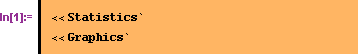``

### Quasi-Monte Carlo Methods

#### Generating of Quasi-Monte Carlo sequences

Aim of the Monte-Carlo method is in the end to explore an unknown result space. The problem with Monte Carlo is that this exploration is random. This means that a specific area in the result space might be explored multiple times other areas are not explored at all. This problem can be seen by visualizing the generated random numbers.

``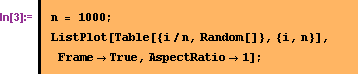``There are clusters in some areas other areas are white. An alternative to random numbers are determistic number sequences which explore the result space systematicly. An example for such low discrepancy sequences are van-der Corput and Halton sequences. Following Ötken (1999) these sequences can be implemented in Mathematica as

``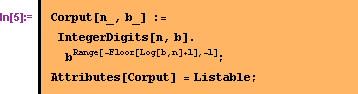``

The first argument defines which element of the row will be calculated the second argument defines the basis to which the sequence is generated. Extensions of the van-der-Corput sequences are the Halton sequences (For further details on these sequences see Ötken (1999)).

``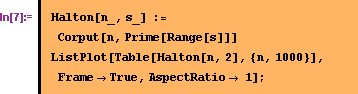``#### Using the low discrepancy sequences

The simplest method to use the low discrepancy sequences is to overwrite the build in random generator of Mathematica.  The sequence can be generated once up front as an array.

``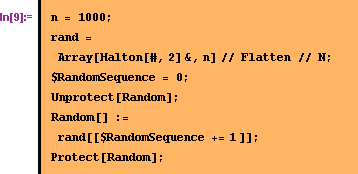``

Each call to Random returns a number of the sequence. The global variable \$RandomSequence makes the necessary bookkeeping to assure that the returned number is always the next within the sequence.

````
``
``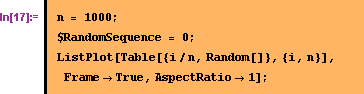``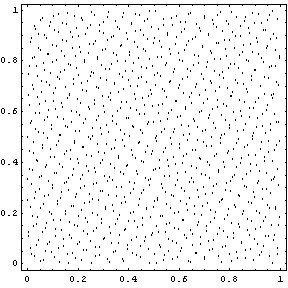#### Using the low discrepancy sequences together with the normal distribution

Mathematica uses a variant of the Box-Muller method to generate normal distributed random numbers. Within this method two equally distributed random numbers are used. Therefore a sequence of 2000 numbers is needed to generate 1000 normal distributed random numbers.

`````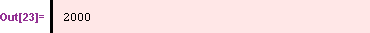`

The advocated approach does not always work with RandomArray. The reason for this is that Mathematica automaticly compiles the function connected with RandomArray when the package is loaded (see the comments from the original developer in the package Statistics`NormalDistribution`). This problem can be circumvated if the build-in random generator is overwritten before the package is loaded.

#### Using the low discrepancy sequences together with the normal distribution

Following the described approach for low discrepancy sequences the implementation of a simple option is the same as with the normal Monte-Carlo method.

````
``

#### Undoing the changes to Random[]

If it is necessary to recover the build-in random generator of Mathematica within a session the overwriting can be undone with the following commands.

````

### Literature

Boyle, Philm P., 1977, Options: A Monte Carlo Approach, in: Journal of Financial Economics, 4/1977, p.141-158.
Clewlow, L./Strickland, C., 1998, Implementing Derivatives Models, John Wiley & Sons Ltd.
Ötken, Giray, 1999, Quasi-Monte Carlo Methods in Option Pricing, in: Mathematica in Education and Research, Vol. 8, No. 3-4, p. 52-57.
Shaw, William: Modelling Financial Derivatives with Mathematica, Cambridge 1998.

Converted by Mathematica      October 1, 2000